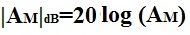﻿ How to Calculate the Midband Gain of a Transistor Circuit# How to Calculate the Midband Gain of a Transistor Circuit

The formula to calculate the Midband Gain, AM, of a transistor circuit is:However, in order to calculate this midband gain, complete AC analysis must be done.

Below is a transistor circuit which we will find the midband gain for:Below is the equivalent AC Equivalent Circuit of the schematic above: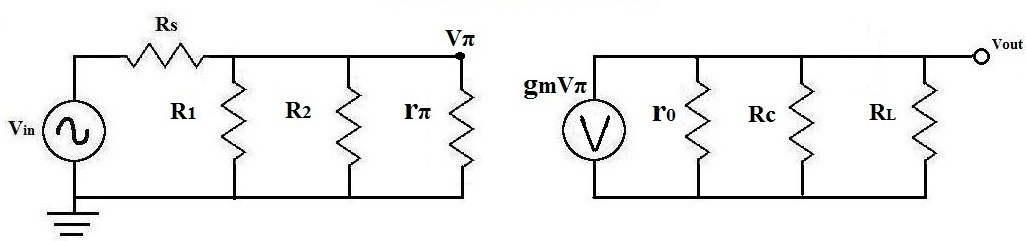AC Analysis

Solve R1||R2 (which is RB)

The first thing to do is solve for RB:Solve for RB|| RB' Next, after you get the value for RB, solve for RB', which is RB||rπ:Solve for Output Resistance RL'
Next, we solve for the output resistance of the transistor circuit, RL', which equal to r0 || RC || RL.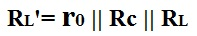Solve for Vπ
Next, we solve for Vπ, which is the voltage that drops across rπ. This is important for other calculations in the AC analysis.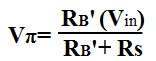Once we have these calculations, we can reduce the above circuit to the one below:Now we can solve for VOUT and then the gain of the transistor, AM: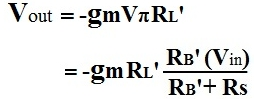We now solve for the midband gain of the transistor circuit, AM: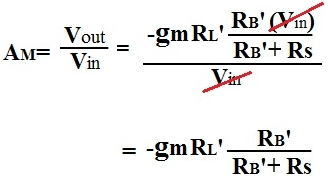The Midband Gain calculated in dB: